1. BW-BS balls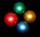Adam has a full box of balls that are large or small, black or white. Ratio of large and small balls is 5:3. Within the large balls the ratio of the black to white is 1:2 and between small balls the ratio of the black to white is 1:8 What is the ratio of
2. Park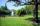In the newly built park will be permanently placed a rotating sprayer irrigation of lawns. Determine the largest radius of the circle which can irrigate by sprayer P so not to spray park visitors on line AB. Distance AB = 55 m, AP = 36 m and BP = 28 m.
3. KLMNIn the trapezoid KLMN is given this informations: 1. segments KL and MN are parallel 2. segments KL and KM has same length 3. segments KN, NM and ML has same length. Determine the size of the angle KMN.
4. Pigs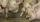Pigs are feed by beet.Beet feed containing 12% dry solids, which is 0.72% of digestible crude protein. How much beet must beconsumed in one month (30 days), if the weight of digestible crude protein contained in a daily dose of beet was 0.912 kg?
5. Exam average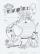Average of marks that have on the certificates students of 8.A class in mathematics is exactly 2.45. If we did not add 1 and 3 of sutdents Michael and Alena, who arrived a month ago, it would average exactly 2.5. Determine how many students have class 8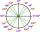What is greater angle? -0.7 radians or -0.7π radians?
7. Rectangle - sides 3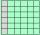If in the rectangle ABCD we enlarge the side a by 5 cm and decrease the side b by 2 cm, the rectangle area will be reduced by 5 cm². When we decrease the length of the side a by 4 cm and and at the same time we increase the length of side b by 3 cm we inc
8. RT 11Calculate the area of right tirangle if its perimeter is p = 45 m and one cathethus is 20 m long.8.3 meters long ladder is leaning against the wall of the well, and its lower end is 1.2 meters from this wall. How high from the bottom of a well is the top edge of the ladder?
10. TilesFrom how many tiles 20 cm by 30 cm we can build a square of maximum dimensions, if we have maximum 881 tiles.
11. Alloy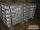The first alloy is a mixture of two metals in the ratio 1:2, the second is a mixture of same metals in the ratio 2:3. At what ratio we have these two alloys put into the furnace to obtain a new metal alloy with ratio 17:27? (All three ratios correspond to
12. DiamondRhombus has side 17 cm and and one of diagonal 22 cm long. Calculate its area.
13. Bouquet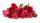Gardener tying bouquet of flowers for 8 and none was left. Then he found that he could tying bouquet of 6 flowers and also none was left. How many have gardener flowers (minimum and maximum) if they had between 50 and 100 flowers?
14. SegmentsLine segments 62 cm and 2.2 dm long we divide into equal parts which lengths in centimeters is expressed integer. How many ways can we divide?
15. Percentage reduction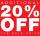Reducing the unknown number by 19% get number 700.7 Determine unknown number.
16. Roots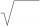Calculate the square root of these numbers:
17. Liters in cylinderDetermine the height at which level 24 liters of water in a cylindrical container having a bottom diameter 36 cm.
18. Logik game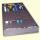Letter game Logik is a two player game, which has the following rules: 1. The first player thinks five-letter word in which no letter is not repeated. 2. The second player writes a five-letter word. 3. The first player answers two numbers - the first numb
19. Stock market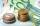Simon one day decided to invest € 62000 to the stock market. After six months he invested July 25 stock markets fell by 47%. Fortunately for Simon from July 25 5 to October 25 his shares have risen by 39%. Simon is then: ?
20. Midpoint of segmentPoint A has coordinates [-16; 23] and the midpoint of the segment AB is the point [2; 12]. What are the coordinates of point B?

Do you have an interesting mathematical word problem that you can't solve it? Submit math problem, and we can try to solve it.

We will send a solution to your e-mail address. Solved examples are also published here. Please enter the e-mail correctly and check whether you don't have a full mailbox.

Please do not submit problems from current active competitions such as Mathematical Olympiad, correspondence seminars etc...